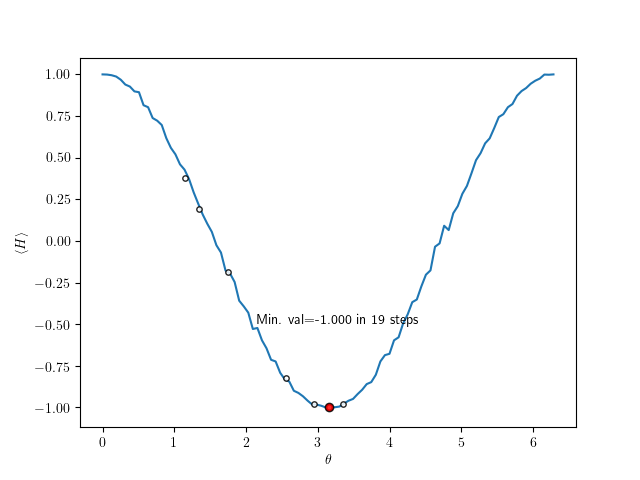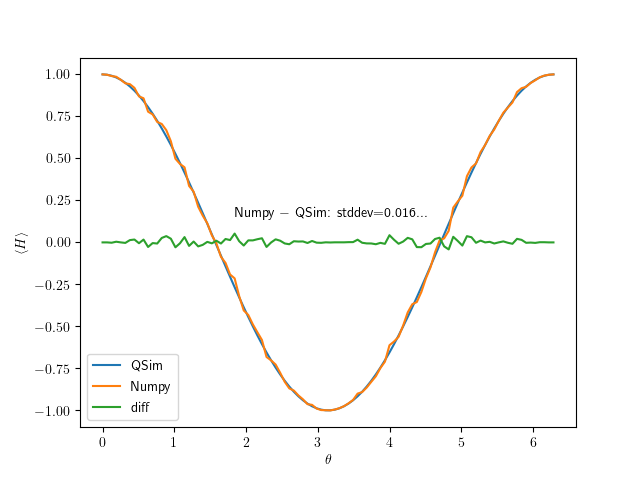# QNLP

Quantum Natural Language Processing

# VQE usage example

Here we demonstrate the usage of the Python-bindings atop our library and use Intel-QS to perform a simple VQE example, and compare the results with an exact simulation using Numpy. This example will be available as the Jupyter notebook VQE.ipynb.

# Variational quantum eigensolver example

Following the approach for VQE given here https://grove-docs.readthedocs.io/en/latest/vqe.html, we can consider the expectation value of the $\sigma_z$ operator, in which case the minimum is the eigenvalue -1.

We construct the ansatz for our state as $$\vert \psi(\theta) \rangle = U(\theta) \vert 0 \rangle,$$ where $U(\theta)$ is an operator controlled by a parameter, $\theta$. For comparison with the example given earlier, we consider

$$U(\theta) = R_y(\theta) = \exp\left(\frac{i\theta \sigma_y}{2}\right) = \begin{pmatrix} \cos(\theta/2) & -\sin(\theta/2) \newline \sin(\theta/2) & \cos(\theta/2) \end{pmatrix}.$$

Following https://dkopczyk.quantee.co.uk/vqe/ our Hamiltonian for this problem, $\sigma_z$, can be decomposed into projective measurements in the $z$-basis as

$$\sigma_z = \begin{pmatrix} 1 & 0\newline 0 & -1 \end{pmatrix} = \vert 0 \rangle \langle 0 \vert - \vert 1 \rangle \langle 1 \vert = \begin{pmatrix} 1 & 0\newline 0 & 0 \end{pmatrix} - \begin{pmatrix} 0 & 0\newline 0 & 1 \end{pmatrix}$$

As such, the entire problem can be solved by measuring the state following a rotation, and tracking whether the result gives a 0 (state $\vert 0 \rangle$ with eigenvalue 1) or a 1 (state $\vert 1 \rangle$ with eigenvalue -1). The difference of their probabilities will give the resulting expectation value of the operator.

Using our bindings for Intel-QS, the example is run as follows. First, we set up the plotting environment.

%matplotlib notebook
from matplotlib import rc
rc('text', usetex=True)
import matplotlib.pyplot as plt


Next, we import necessary packages. We include scipy.optimize.minimize as part of the classical optimisation routine to find the lowest eigenvalue.

from PyQNLPSimulator import DCMatrix, GateOps, PyQNLPSimulator
from PyQNLPSimulator import GateOps
import numpy as np
from scipy.optimize import minimize


We choose the size of our quantum circuit (at least 1 qubit for this example), and the number of sample to take we calculating expectation values.

num_qubits = 2
use_fusion = False
sim = PyQNLPSimulator(num_qubits, use_fusion)
num_exps = 2000
normalise = True
gops = GateOps(sim)


We now define the methods to perform our experiments.

• collapse_val: This returns 0 or 1, the result of measuring the state in the Z-basis. By sampling this we can build a distribution of the probability of state occupation.
• expec_val: Using the probabilities determined from sampling collapse_val, we can infer the expectation value for the given sample Hamiltonian.
def collapse_val(sim, theta):
"""
Return the measurement value of the state following rotation by theta
"""
sim.initRegister()

sim.applyGateRotX(0, theta)

val = sim.applyMeasurementToRegister(, normalise)
return val

def expec_val(theta, sim):
"""
Calculate the averaged result to yield the expectation value of the given problem
"""
p0 = 0
p1 = 0
for i in range(num_exps):
tmp = collapse_val(sim, theta)
if tmp == 0:
p0 += 1
else:
p1 += 1
return (p0 - p1) / num_exps


To view the progress of the optimiser, we add a callback routine to output the parameter value and subsequent eigenvalue.

def cb(x):
global g_vals
g_vals.append([x, expec_val(x, sim)])


We can generate a run script to create a sample expectation value over the range $\theta \in [0, 2\pi)$

def run(sim):
"""
Generate sampled values over the range of angle from 0 to 2pi
"""
vals = {}
for v in np.linspace(0, 2*np.pi, 100):
val = expec_val(v, sim)
vals.update({v : val})
return vals


Next, we plot the result and use the Scipy minimisation method to find the lowest eigenvalue with calls to the expec_val method. The number of samples to find this minimum value is plotted, alongside the value itself.

g_vals = []
vals = run(sim)
plt.figure()
plt.plot(list(vals.keys()), list(vals.values()), zorder=1)
min_val = minimize(expec_val, 1.0, args=(sim), method="nelder-mead", callback=cb)

#Intermediate steps of solver
x_val = [i for i in g_vals]
y_val = [i for i in g_vals]
plt.scatter(x_val, y_val, c='w', alpha=0.85, s=15, edgecolors="k", zorder=2)
plt.xlabel(r'$\theta$')
plt.ylabel(r'$\langle H \rangle$')

#Minimum found
plt.scatter(min_val.x, min_val.fun, c='r', edgecolors="k", zorder=3, alpha=0.85)
plt.text(min_val.x-1.0, min_val.fun+0.5, "Min. val={:1.3f} in {} steps".format(min_val.fun, min_val.nit))# VQE in numpy

For comparison, we can expclitly calculate the values in Python by defining the appropriate matrix and vector types.

def gen_state(theta):
return np.dot(gops.RY(theta), np.array([,])), np.dot( np.array([[1,0]]), gops.RY(-theta) )

gen_state(np.pi)
num_samples = 100

ham = np.array([[1,0],[0,-1]])
res_map = {}

for i in np.linspace(0, 2*np.pi, 100):
s1,s1ct = gen_state(i)
res = 0
for k in range(num_samples):
res += np.asarray(s1ct*ham*s1).flatten()
res /= num_samples
res_map.update({i : res})

plt.figure()
sdev = np.std(np.array(list(vals.values())) - np.array([np.real(i) for i in res_map.values()]))
plt.plot(list(res_map.keys()), [np.real(i) for i in res_map.values()], label="QSim")
plt.plot(list(vals.keys()), list(vals.values()), label="Numpy")
plt.plot(np.array(list(vals.keys())), np.array(list(vals.values())) - np.array([np.real(i) for i in res_map.values()]), label="diff")
plt.text(np.pi-1.3, 0.15, "Numpy $-$ QSim: stddev={:1.3f}...".format(sdev))
plt.xlabel(r'$\theta$')
plt.ylabel(r'$\langle H \rangle$')
plt.legend()Plotting both results show a high degree of similarity between both results. The quantum simulator result, being probability, obviously has some variation in its output.

Last updated on 1 Mar 2020
Published on 1 Mar 2020
Edit on GitHub